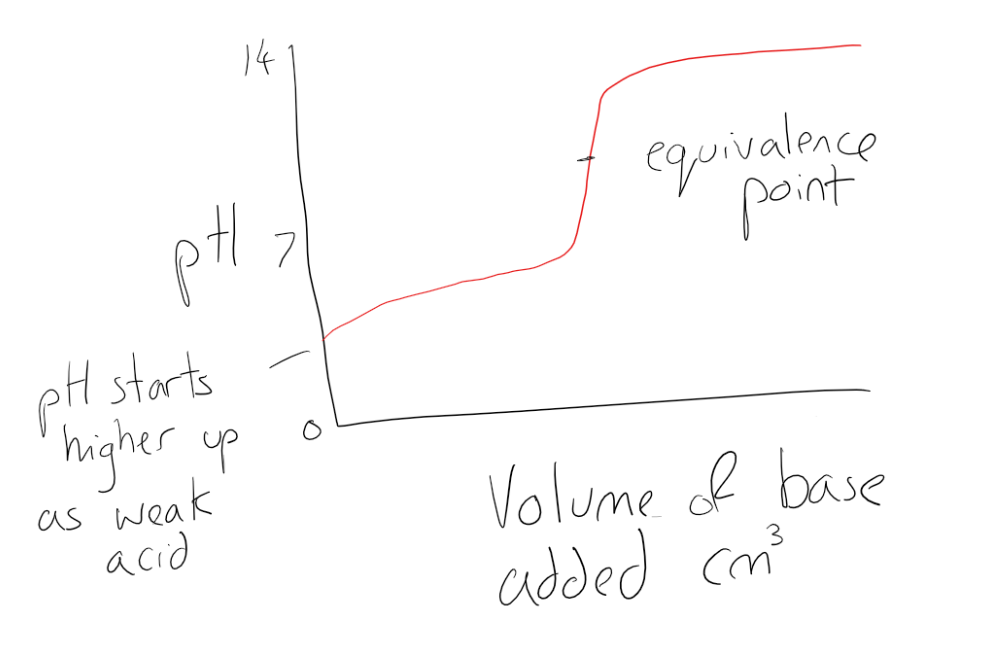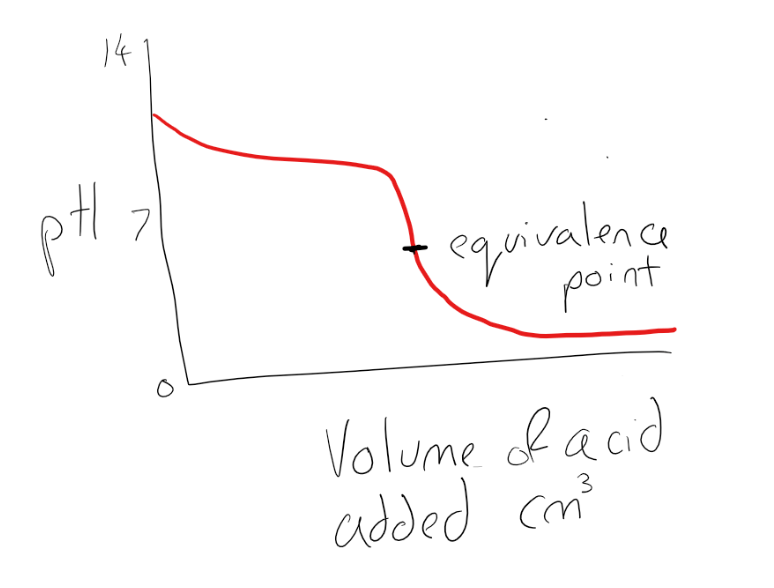Complimentary 1-hour tutoring consultation

MCAT Content / Titration / Interpretation Of The Titration Curves

### Interpretation of the Titration Curves

Topic: Titration

A titration curveis a graphical representation of the pH of a solution during a titration

Titration curves can be used to track the reaction of acids and bases and at the equivalence point, the amount of titrant added to neutralize the analyte can be recorded. This varies for different types of acids and bases due to their relative strength.

Strong Acid-Strong BaseA strong acid-strong base titration is performed using a phenolphthalein indicator. Phenolphthalein is chosen because it changes color in a pH range between 8.3 – 10. It will appear pink in basic solutions and clear in acidic solutions. Neutralization occurs at pH 7.0 as there are equal moles of acid and base. An example of a strong acid and strong base is the addition of hydrochloric acid to sodium hydroxide to form sodium chloride and water (HCl + NaOH → HCl + H2O).

Weak Acid-Strong BaseA weak acid will react with a strong base to form a basic (pH > 7) solution. Neutralization does not occur at pH 7.0 this is due to the production of the conjugate base during the titration. The resulting solution is slightly basic. Instead, the equivalence point is where the number of moles of weak acid and base is equal. In a weak acid and strong base titration, the equivalence point and the endpoint can be different due to the formation of the conjugate base. An example of a weak acid-strong base titration involves reacting ethanoic acid with sodium hydroxide to form sodium ethanoate and water:

NaOH + CH3COOH → CH3COONa+ + H2O

Weak Base-Strong AcidIn the case of titrating the acid into the base for a strong acid-weak base titration, the pH of the base will ordinarily start high and drop rapidly with the additions of acid. In strong acid-weak base titrations, the pH at the equivalence point is not 7, but below it. This is due to the production of a conjugate acid during the titration; it will react with water to produce hydronium (H3O+) ions. An example of a strong acid–weak base titration is the reaction between ammonia and hydrochloric acid:

NH3 + HCl → NH4+ + Cl

The ammonium formed reacts with water to produce the hydronium ion making the equivalence point lower than pH 7.0:

NH4+ H2O -> H3O+ + NH3

Practice Questions

MCAT Official Prep (AAMC)

Section Bank C/P Section Passage 6 Question 44

Key Points

• Titration curves show the progress of a titration as the volume of the titrant is added to the analyte and the pH changes.

• Different combinations of strong/weak acids and strong/weak bases produce different shaped titration curves.

• The equivalence point is not always at pH 7.0, but is the point at which the moles of titrant is equal to that of analyte.

Key Terms

Equivalence point: The point at which the equivalent number of moles of a base have been added to an acid.

Endpoint: The term given to the color change of an indicator when an acid and base react.

Titration curve: a plot showing the change in pH of the solution in the conical flask as the titrant is added from the burette to the analyte.

Titrant: The acid or base in the burette that has a known concentration.

Analyte: The acid or base which has an unknown concentration.

Neutralization: A reaction when an acid and a base react to form water and a salt, and involves the combination of H+ ions and OH ions to generate water.

Strong acid: An acid that is completely dissociated or ionized in an aqueous solution.

Strong base: A base that is completely dissociated in an aqueous solution.

Weak acid: An acid that partially dissociates into its ions in an aqueous solution or water.

Weak base: A weak base is a chemical base that does not ionize fully in an aqueous solution.

Billing Information